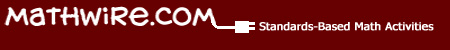Discrete Math## Featured Activities: Pascal's Triangle Activities

These seasonal activities were developed to provide students opportunities to analyze and learn the patterns of Pascal's Triangle.   Through repeated exposure to this important mathematical pattern, students will build understanding of the pattern and will more easily relate its use to mathematical problems they meet in the future.

• SeePascal's Pumpkins, based on Rutger's Discrete Math Program activity.
• SeePascal's Ghosts, based on Rutger's Discrete Math Program activity.
• SeePascal's Bats
• SeePascal's Penguins 1.
• SeePascal's Penguins 2.
• SeePascal's Hearts.
• SeeShamrock Paths

## Discrete Math

These activities help students use organized lists and systematic counting to solve combination problems.   Map coloring and networks are also discrete math problems that students can relate to real-world applications.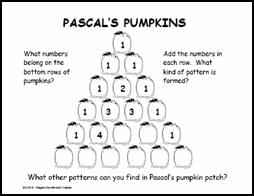### Pascal Patterns & Paths

•Pascal's Pumpkins   encourages students to look for patterns in Pascal's Triangle.   The handout develops awareness of this important mathematical pattern through this timely seasonal activity.   Students who take higher math courses will meet Pascal again in many different applications, including probability.   This handout is an outgrowth of the Rutgers Discrete Math Institute.
•Pascal's Ghosts: How Many Ghosts Do You See?   encourages students to apply the patterns in Pascal's Triangle.   A teacher instructional plan with mathematical background, answer and challenge is included to explain how to present this problem which is also an outgrowth of the Rutgers Discrete Math Institute.   A recording sheet was also included as part of the teacher packet so that students are able to record all different solutions in a "systematic" way, which is a goal of discrete mathematics.
•Pascal's Bats challenges students to identify and use the patterns in Pascal's Triangle to complete the unfinished row and to add the next row to the pattern.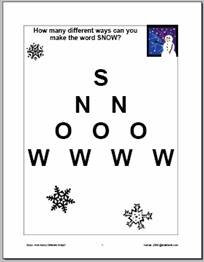•Pascal's Penguins - 1 introduces primary students to a small version of Pascal's Triangle in this simple patterning activity.
•Pascal's Penguins - 2 introduces a larger version of Pascal's triangle and encourages students to identify the different patterns within the triangle and use these patterns to fill in the missing penguin numbers.
•How Many Different Ways Can You Make Snow? requires students to find all of the paths to make SNOW.   Use the recording worksheet to assess student use of systematic counting techniques to find all of the ways and relate them to the appropriate row of Pascal's triangle. [PDF]
•Winter Paths requires students to use systematic counting techniques to find all of the paths that spell WINTER and relate the solution to the appropriate row of Pascal's triangle. [PDF]•Pascal's Hearts introduces students to Pascal's Triangle in this simple Valentine's Day patterning activity.
•Shamrock Paths introduces students to Pascal's Triangle in this simple St. Patrick's Day patterning activity.

### Combinations: Organized Counting

•Houses in a Row challenges students to figure out how many different color combinations are possible for a row of houses, if no two houses can be painted exactly alike. Use large color squares and triangles to model this problem for students as an introduction to combinations.
•Stoplights challenges students to figure out how many different stoplights they can create with 3 different color linking cubes if each stoplight must have one cube of each color. Use linking cubes so that students can assemble all possible stoplights. You may choose to have students use any three different colors so that each student pair has enough linking cubes to assemble all possible combinations using one of each color to create the stoplight.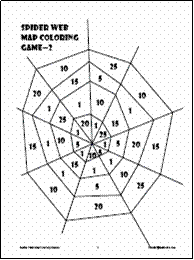### Map Coloring

•Spider Web Map Coloring Games: this 2-4 player game challenges students to apply map coloring rules to win the most points by coloring in sections of the spider web on each dice toss. Students toss a special die (1-1-1-2-2-lose turn) to find out how many sections they may color in on that turn. The game ends when no player can color another section according to map coloring rules.### Codes

•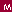Data Investigations: Codemaking and Codebreaking: this page investigates mathematical codes and features links to historical and current applications of mathematical codemaking and codebreaking. This is a critical issue for the security of information sent via e-mail or the internet. The page also contains "Crypto Lists" which challenge students to analyze letter frequency to decode these related lists.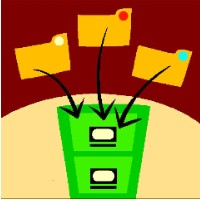• See moreDiscrete Math Templates: insert in sheet protectors for student use with dry erase markers or for teacher use as overhead transparencies.
• SeeProblem Solving Resources for open-ended assessments that involve discrete math.
• See allDiscrete Math & Logic Games.
• See moreDiscrete Math Links.
• See allEnrichment Activities for discrete math.